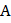# The elevator shown in figure is descending with an acceleration of. The mass of the blockkg. Find the force (in Newton) exerted by the blockon the block## Question ID - 153893 :- The elevator shown in figure is descending with an acceleration of. The mass of the blockkg. Find the force (in Newton) exerted by the blockon the block3537

(4)

Letapply a forceonThenalso applies an opposite forceonas shown in figure

ForN

Next Question :

A block, of weight, slides down an inclined planeof slopeat a constant velocity, while the plank, also of weight, rests on top of. The plankis attached by a cord to the top of the plane. The coefficient of kinetic frictionis the same between the surfacesandand betweenand. Determine the value of